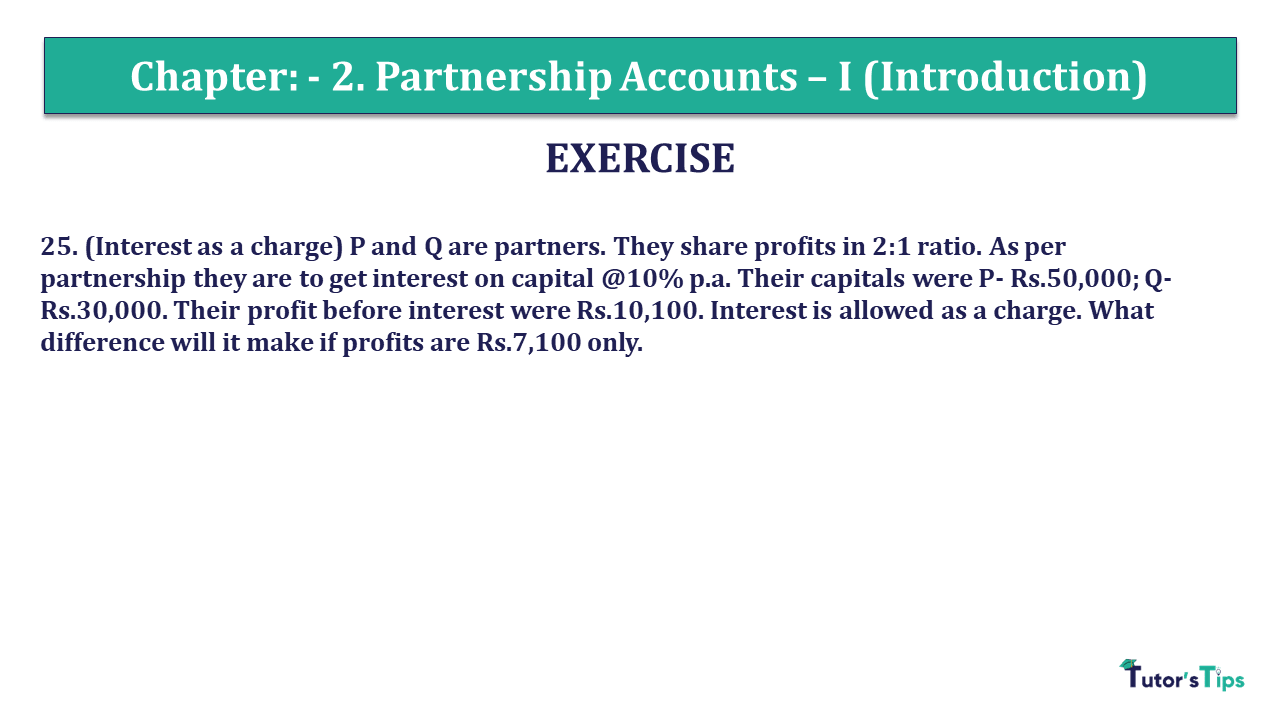# Question 25 Chapter 2 of +2 Part-1 – USHA Publication 12 Class Part – 1Q-25 - CH-2 - Usha +2 Book 2018 - Solution

Question 25 Chapter 2 of +2-Part-1

25. (Interest is a charge) P and Q are partners. They share profits in 2:1 ratio. As per the partnership, they are to get interested on capital @10% p.a. Their capitals were P- Rs.50,000; Q- Rs.30,000. Their profit before interest was Rs.10,100. Interest is allowed as a charge. What difference will it make if profits are Rs.7,100 only?

### The solution of Question 25 Chapter 2 of +2 Part-1: –

 Calculation of net profit Particulars B a) If profits are Rs.10,100: Profit before interest 10,100 Less: Interest on Capital @ 10% – A (5,000) Interest on Capital @ 10% – B (3,000) Net Loss 2,100

 Calculation of distribution of profit Particulars A B Net Profit of Rs.2,100 being distributed in 2:1 ratio 1,400 700

 Calculation of net profit Particulars B a) If profits are Rs7,100: Profit before interest 7,100 Less: Interest on Capital @ 10% – A (5,000) Interest on Capital @ 10% – B (3,000) Net Loss 900

 Calculation of distribution of profit Particulars A B Net loss of Rs.900 being distributed in 2:1 ratio (600) (300)

Note: When interest is allowed as charge, it is irrespective of the quantum of profits and is shown in Profit and Loss a/c.

Comment if you have any questions.

Also, Check out the solved question of previous Chapters: –